# Convolutional Neural Network Explained SO EASY

Recently, deep learning is being applied to numerous areas and it shows amazing performance especially in the computer vision area. Computer vision is an area that interprets the vision information and utilizes it by computers. You can do image classification, object detection, optical character recognition, and many other fascinating computer vision tasks using deep learning.

In this post, let's take a look at the Convolutional Neural Network (so-called CNN) which is used for deep learning computer vision tasks.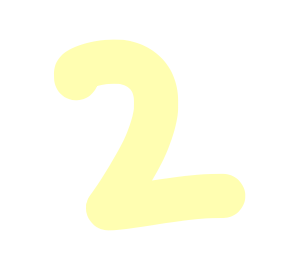Let's say there is handwritten image data representing "2".
This data is saved in the computer as a 2-dimensional array like below.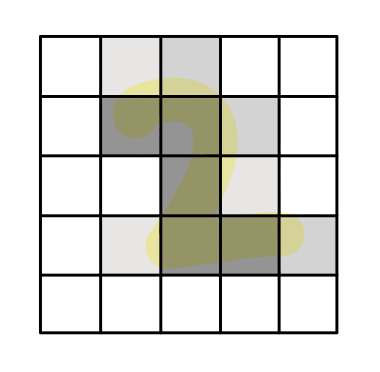When you say 10-megapixel resolution about your cell phone camera, it means there are 10 million cells in a 2-dimensional array on the picture taken by that camera (with maximum resolution setting.) Each cell is called "pixel". So our example data is composed of 25 pixels (5 X 5).

Each pixel has a value that represents the brightness of its location.
In the case of black and white image data, 0 means black and 1 means white.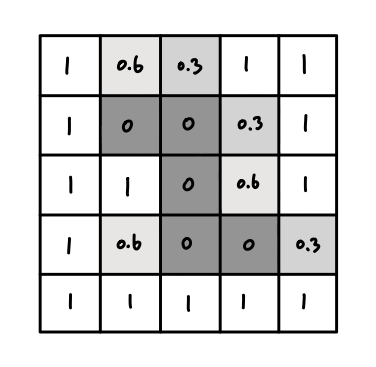By this logic, the data of "2" is a 2-dimensional array that has 25 values of brightness. To make it simple, I compose it with only four values - 0, 0.3, 0.6, 1.

Now let's add some transformations here.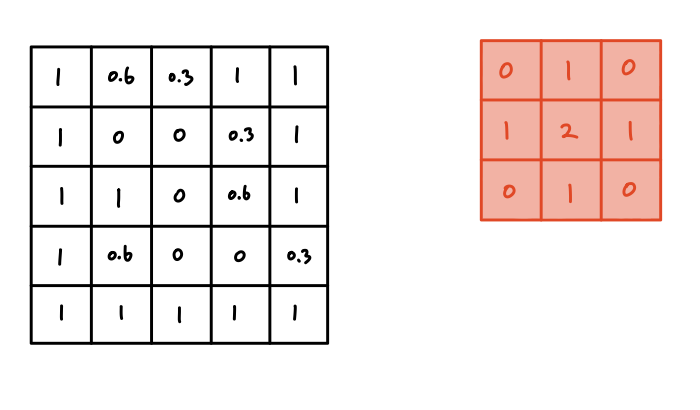We will transform the data on the left using the 3 X 3 size array on the right. This red array is called a filter.

Transformation is simple.
The filter slides on the data, multiplying the values on the same pixel. You can understand this as a weighted sum operation - summing data values after multiplying each value's weight.

The result of the operation in the first slide is as above.
Let's go line by line.

1. Multiplying the first row of the data [1,  0.6,  0.3]
and the first row of the filter [0,  1,  0]
results in [0,  0.6,  0]   (1 x 0 = 0, 0.6 x 1 = 0.6, 0.3 x 0 = 0)

2. Multiplying the second row of the data [1,   0,   0]
and the second row of the filter [1,    2,   1]
results in [1,   0,   0]

3. Multiplying the third row of the data [1,    1,   0]
and the first row of the filter [0,   1,   0]
results in [0,   1,   0]

4. Summing all of those values gives 2.6. This is the result of this operation.

The next operation starts after the filter slides one cell.
The values in the filter are fixed, and they will be multiplied by the values in the same pixels of the data. Then, we save this second result next to the previous result.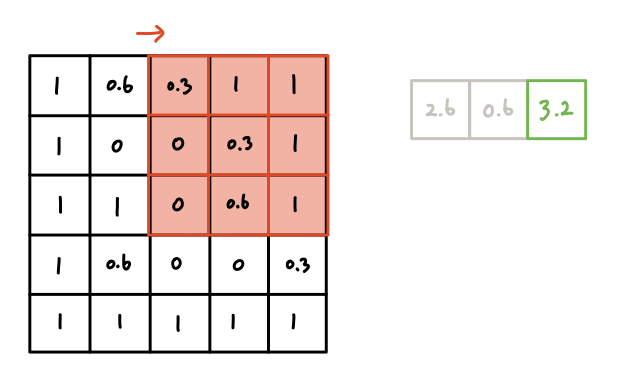And so on.
Now the filter reached the right end of the data. What can we do?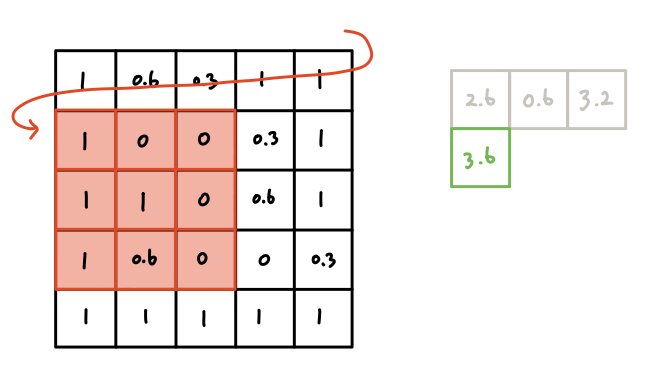It goes one cell down and starts from the left again.
When you finish this process until the end of the data, you will get the 3 X 3 size result.

Why 3 X 3?
Let's think about the width only. The length of the data is 5 and the length of the filter is 3. So filter does the operation 3 times.* Same as height, so the number of operations becomes 3 X 3, 9.

* If filter slides 2 cells each time, then filter locates only two times. So the size of the result will be 2 X 2, 4. This parameter determines how many cells the filter slides each time is called stride. If you want to make the size of the result the same as the original data, then you can surround the data with zeros before starting the sliding operations. Then the size of the data will be 7 X 7, and the size of the result will be 5 X 5. This trick is called (zero) padding.

This sliding operation is called convolution.*

* This does not have the exact same meaning of convolution in mathematics, but we use it this way in the deep learning field.

Now let's do the same process using another filter. Since the filter has different values this time, the result will be different.

We can keep doing this by using various filters. We will have multiple result arrays of the convolution operations. So what's the meaning of doing this?
The first cell in a result array comes from multiplying the left-top 3 X 3 part of the data and the filter. This means a filter summarized 3 X 3 cells into 1 cell by the convolution operation.

Doing the convolution operations using filters, therefore, generates multiple results which have summarized and interpreted regional information of the original image data. Each pixel of the result arrays contains 9-pixel information - meaning that the computer can see multiple pixel information at the same time with one value.

In other words, the result arrays are the extracted features from the original image data. If you use 5 filters, then you will get 5 new features from the data. Now we get the familiar structure - Neural Networks.

Result array from a convolution operation using a filter is called a feature map. The number of feature maps is called the number of channels as well. If you use 10 filters, then there will be 10 output channels.

Having multiple channels means that there are multiple 2-dimensional arrays, which is identical to having one 3-dimensional array. We used single channel (black and white) data, but the colored image data has 3 channels - Red, Green, Blue channels.

So what kind of values should be in the filters?
Before the era of deep learning, the values in the filter were fixed. Have you ever used "sharpen filter" in a photo editor? The red filter we used earlier is actually almost the same as the sharpen filter. (Try converting 1s to -1)

However, the values are not fixed when it comes to deep learning. The key principle of "learning" is changing the values of the weights into certain directions so that the model makes better predictions. The values in a convolution filter are the weights of a Convolutional Neural Network.

That means, we put some random values into the filters and set how many filters we use in each layer before starting training, but the values in the filters change as the training goes on. By doing so, the values in the filters are getting optimized, more meaningful feature maps are being extracted, so the model makes better predictions.

See, Convolutional Neural Network is also just a Neural Network.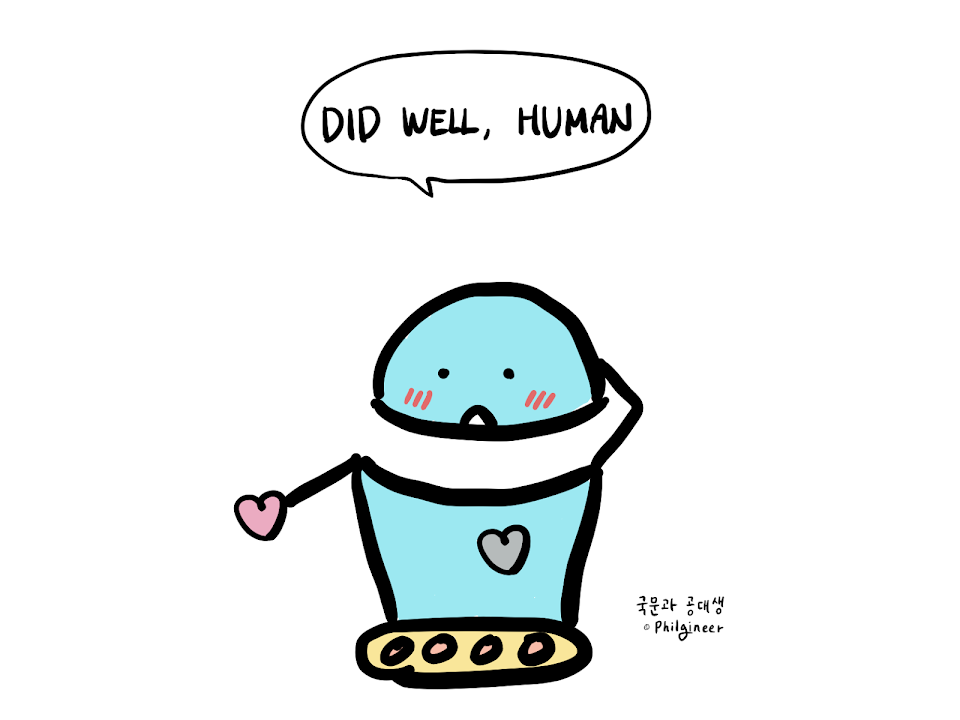Reference for going deeper